# KSEEB Solutions for Class 9 Maths Chapter 1 Number Systems Ex 1.3

In this chapter, we provide KSEEB SSLC Class 9 Maths Chapter 1 Number Systems Ex 1.3 for English medium students, Which will very helpful for every student in their exams. Students can download the latest KSEEB SSLC Class 9 Maths Chapter 1 Number Systems Ex 1.3 pdf, free KSEEB SSLC Class 9 Maths Chapter 1 Number Systems Ex 1.3 pdf download. Now you will get step by step solution to each question.

## Karnataka Board Class 9 Maths Chapter 1 Number Systems Ex 1.3

Question 1.
Write the following in decimal form and say what kind of decimal expansion each has :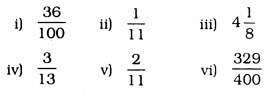(i)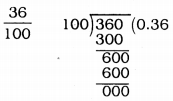Terminating decimal expansion.
(ii)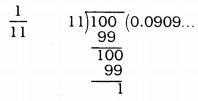Non-terminating decimal expansion.
Undefined control sequence \therefore
(iii)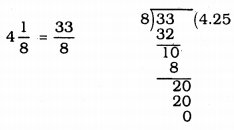∴ Terminating decimal expansion.
(iv)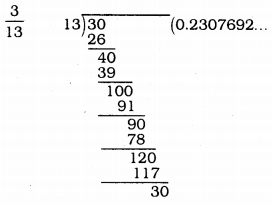0.23069¯¯¯¯¯¯¯¯¯¯¯¯ the bar above the digits indicates the block of digits that repeats.
∴ This is Non-terminating, repeating decimal.
(v)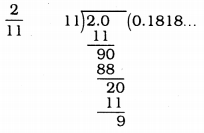Here, 0.18¯¯¯¯¯ is block of digits that repeats.
∴ This is non-terminating, repeating decimal.
(vi)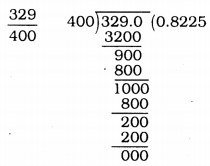329400=0.8225 This is terminating decimal because remainder is zero.

Question 2.
You know that17=0.142857¯¯¯¯¯¯¯¯¯¯¯¯¯¯¯. Can you predict what the decimal expansions of 27,37,47,57,67 doing the long division ? If so, how? (Hint: Study the remainders while finding the value of 17 carefully.).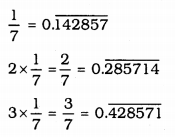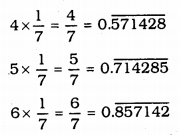Here the first digit in decimal continued repeated numbers afterward.

Question 3.
Express the following in the form pq, where p and q are integers and q ≠ 0. (p, q ϵ Z, q ≠ 0).
(i) 0.6¯¯¯
(ii) 0.47¯¯¯¯¯
(iii) 0.001¯¯¯¯¯¯¯¯
(i) 0.6¯¯¯ = 0.6666……….
Let x = 0.6666………….
∴ x = 0.6666………..
Multiplying both sides by 10,
10x = 6.666…..
10x = 6 + 0.666
10x = 6 + x
10x – x = 6
9x = 6
x=69= form of pq
∴ 0.6¯¯¯=23

(ii) 0.47¯¯¯¯¯ = 0.4777…..
Let x = 0.47¯¯¯¯¯ then x = 0.4777……..
Here digit 4 is not repeating but 7 is repeated.
Let x = 0.4777
Multiplying both sides by 10.
10x = 4.3 + x
10x -x = 4.3
9x = 4.3
Multiplying both sides by 10.
90x = 43
∴ 0.47¯¯¯=4390

(iii) 0.001¯¯¯¯¯¯¯¯ x = 0.001001……….
Multiplying both sides by 1000
1000x = 1.001001
1000x = 1 + x
1000x – x = 1
999x = 1
x=1999
∴ 0.001¯¯¯¯¯¯¯¯=1999

Question 4.
Express 0.99999…….. in the form pq , Are you surprised by your answer ? With your teacher and classmates discuss why the answer makes sense.
Express 0.99999 in the form of pq,
Let x = 0.99999 …
Multiplying by 10.
10x = 9.9999……
10x = 9 + 0.9999…….
10x = 9 + x
10x – x = 9
9x = 9
x=99,x=1=11
∴ (p = 1 & q = 1)
0.99999 …… goes on forever, so there is no gap between 1 and 0.99999 ………… hence they are equal.

Question 5.
What can the maximum number of digits be in the repeating block of digits in the decimal expansion of 117 ? Perform the division to check your answer:
Expansion of 117 in decimal: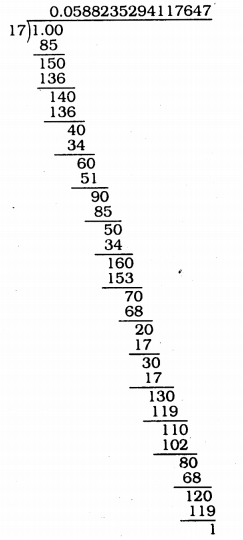Undefined control sequence \therefore
Here repeated numbers are 16.

Question 6.
Look at several examples of rational numbers in the form pq (q≠0), where p
and q are integers with no common factors other than 1 and having terminating decimal representations (expansions). Can you guess what property q must satisfy?
Rational numbers in the form pq (q ≠ 0) where P and q are integers with no common factors other than 2 or 5 or both.
OR
Prime factors of q have powers of 2 and prime factors of q have powers of 5 or both.

Question 7.
Write three numbers whose decimal expansions are non-terminating non-recurring.
Three numbers whose decimal expansions are non-terminating, non-recurring are
i) 0.123123312333
ii) 0.20200200020000
iii) 0.56566566656666

Question 8.
Find three different irrational numbers between the rational numbers 57 & 911 .
57=0.714285¯¯¯¯¯¯¯¯¯¯¯¯¯¯¯911=0.81¯¯¯¯¯
Three irrational numbers between 0.714285¯¯¯¯¯¯¯¯¯¯¯¯¯¯¯ and 0.81¯¯¯¯¯ are :
i) 0.72720720072000………….
ii) 0.7357355735555……………..
iii) 0.760760076000………………

Question 9.
Classify the following numbers as rational or irrational :
(i) 23−−√
(ii) 225−−−√
(iii) 0.3796
(iv) 7.478478
(v) 1.101001000100001…
i) 23−−√ = 4.7958………. This is not terminating or non-recurring decimal.
∴ 23−−√ is an irrational number.
ii) 23−−√ = 15 this is a rational number.
iii) 0.3796 This is rational number, because trminating decimal has expansion.
iv) 0.478478… This is rational number, because decimal expansion is recurring.
v) 0.101001000100001… This is an irrational number because termianting or recruring decimal has no expansion.

All Chapter KSEEB Solutions For Class 9 Maths

—————————————————————————–

All Subject KSEEB Solutions For Class 9

*************************************************

I think you got complete solutions for this chapter. If You have any queries regarding this chapter, please comment on the below section our subject teacher will answer you. We tried our best to give complete solutions so you got good marks in your exam.

If these solutions have helped you, you can also share kseebsolutionsfor.com to your friends.

Best of Luck!!